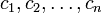# Critical point for a function of multiple variables

## Definition

### Definition in terms of gradient vector

Suppose$f$ is a function of a vector variable$\overline{x}$ with coordinates$x_1,x_2,\dots,x_n$. Suppose$\overline{c}$, with coordinates$c_1,c_2,\dots,c_n$, is a point in the interior of the domain of$f$. We say that$\overline{c}$ is a critical point for$f$ if the gradient vector of$f$ at$\overline{c}$ is either the zero vector or does not exist.

## Facts

### Relation with partial derivatives

The following is true at any point in the interior of the domain of a function:

Partial derivatives with respect to all variables are zero$\implies$ Critical point

The reason is as follows: the gradient vector, if it exists, must be the vector whose coordinates are the partial derivatives. In particular, this means that the gradient vector, if it exists, must be the zero vector. Thus, the gradient vector is either the zero vector or doesn't exist.

However, it's possible for a point in the domain of a function to be a critical point but to not have all partial derivatives equal to zero. This is because the gradient vector may fail to exist even though the partial derivatives do exist.

We can strengthen the result a bit as follows:

Partial derivatives with respect to all variables are zero or any of the partial derivatives is undefined$\implies$ Critical point

### Relation with partial derivatives and directional derivatives

We can build a little more on the previous result and state that, at any point in the interior of the domain of a function:

Directional derivatives in all linear directions are zero$\implies$ Partial derivatives with respect to all variables are zero$\implies$ Critical point

We can also say that:

Directional derivatives in all linear directions are zero or any of the directional derivatives is undefined$\implies$ Critical point